# Ex. 4 A water treatment plant with a maximum daily flow of 3 m/s (1.5 times...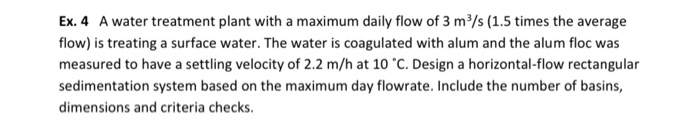Ex. 4 A water treatment plant with a maximum daily flow of 3 m/s (1.5 times the average flow) is treating a surface water. The water is coagulated with alum and the alum floc was measured to have a settling velocity of 2.2 m/h at 10 °C. Design a horizontal-flow rectangular sedimentation system based on the maximum day flowrate. Include the number of basins, dimensions and criteria checks.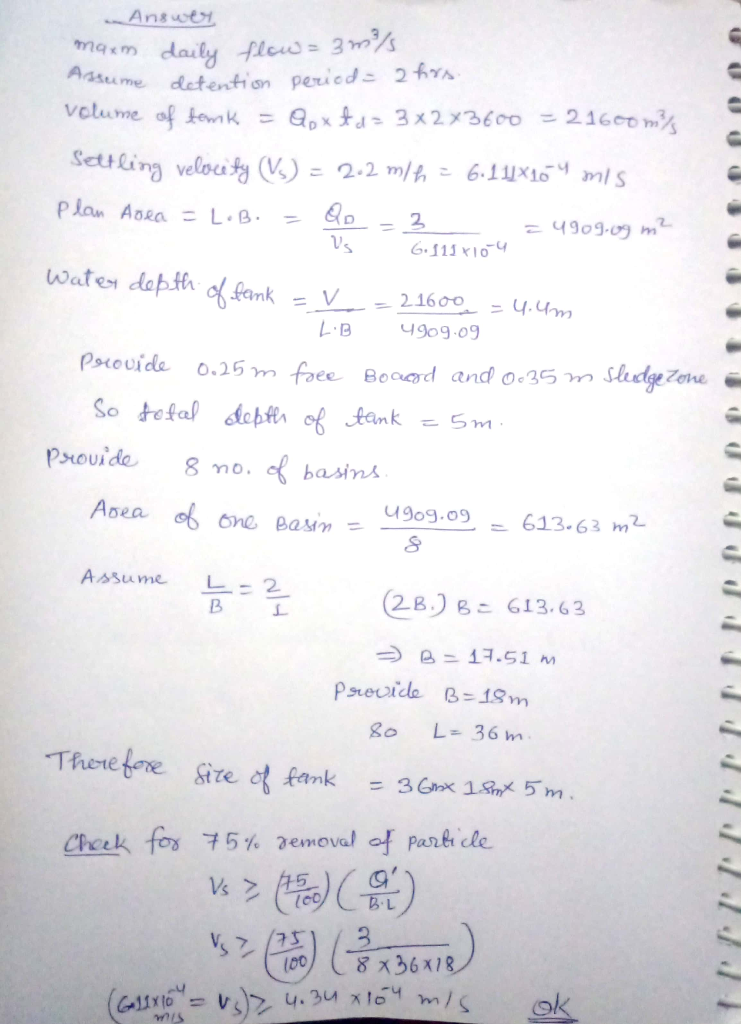#### Earn Coin

Coins can be redeemed for fabulous gifts.

Similar Homework Help Questions
• ### A water treatment plant is processing water at a flow rate of 1.5 m/s using three parallel treatm...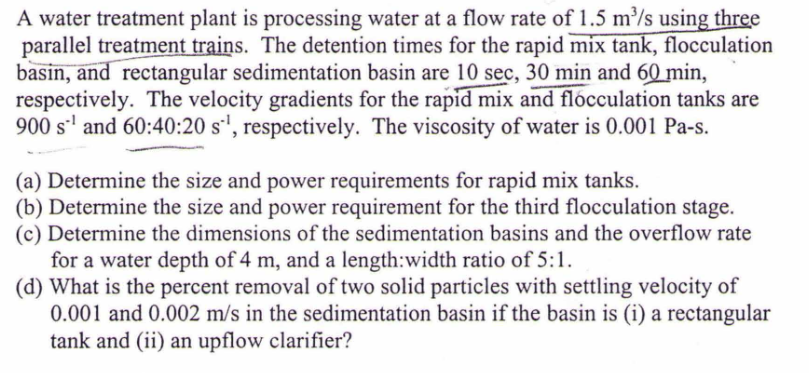A water treatment plant is processing water at a flow rate of 1.5 m/s using three parallel treatment trains. The detention times for the rapid mix tank, flocculation basin, and rectangular sedimentation basin are 10 sec, 30 min and 60 min, respectively. The velocity gradients for the rapid mix and flocculation tanks are 900 s and 60:40:20 s1, respectively. The viscosity of water is 0.001 Pa-s. (a) Determine the size and power requirements for rapid mix tanks. (b) Determine the...

• ### Any tips or pointers? characteristics: Maximum daily flow 3 ms Average daily flow 2 m'/s Coagulant...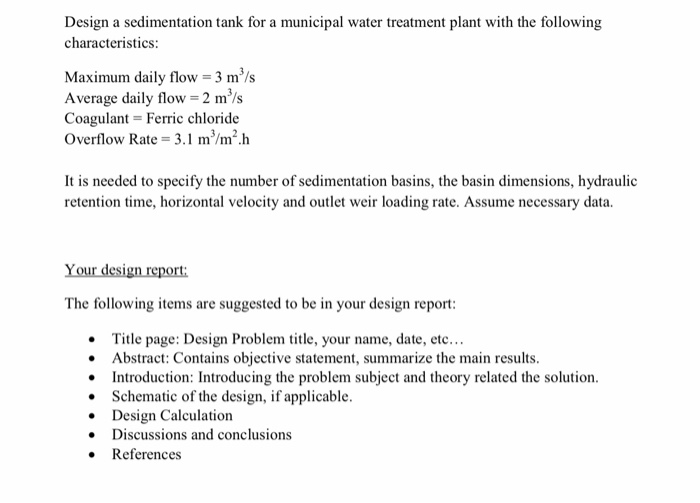Any tips or pointers? characteristics: Maximum daily flow 3 ms Average daily flow 2 m'/s Coagulant Ferric chloride Overflow Rate 3.1 m/m2h It is needed to specify the number of sedimentation basins, the basin dimensions, hydraulic retention time, horizontal velocity and outlet weir loading rate. Assume necessary data. The following items are suggested to be in your design report Title page: Design Problem title, your name, date, etc... Abstract: Contains objective statement, summarize the ma Introduction: Introducing the problem subject...

• ### Water and Wastewater Treatment SP-WATER TREATMENT PLANT SEDIMENTATION BASIN Design a rectangular, horizontal flow sedimentation process...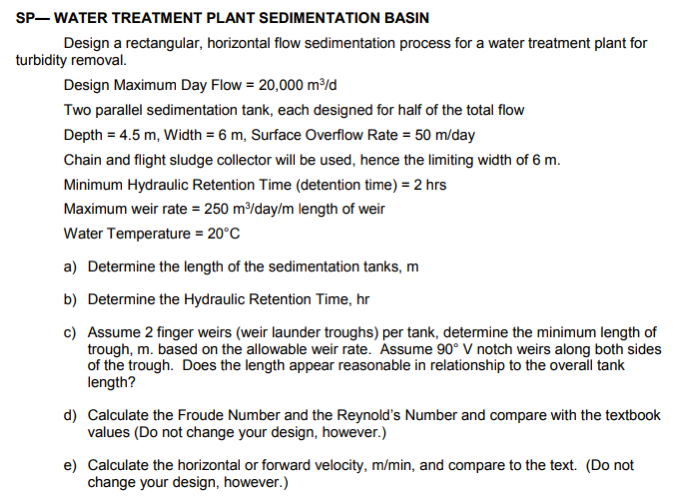Water and Wastewater Treatment SP-WATER TREATMENT PLANT SEDIMENTATION BASIN Design a rectangular, horizontal flow sedimentation process for a water treatment plant for turbidity removal Design Maximum Day Flow-20,000 m2ld Two parallel sedimentation tank, each designed for half of the total flow Depth 4.5 m, Width 6 m, Surface Overflow Rate 50 m/day Chain and flight sludge collector will be used, hence the limiting width of 6 m. Minimum Hydraulic Retention Time (detention time) 2 hrs Maximum weir rate 250 m/day/m...

• ### A drinking water treatment plant is designed with three sedimentation basins in parallel. Each basin is...

A drinking water treatment plant is designed with three sedimentation basins in parallel. Each basin is designed with an overflow rate of 1,200 gpd/ft2 (gallons per day per square foot). (“In parallel” means that the flow is split evenly between the basins as shown below.) A. If the plant flow is 20 mgd (million gallons per day), find the plan area of each basin in square feet. B. Calculate the dimensions of the basins (length and width in feet) if...

• ### 1. The design flow for a water treatment plant (WTP) is 1 MGD (3.8x103 m'/d). The...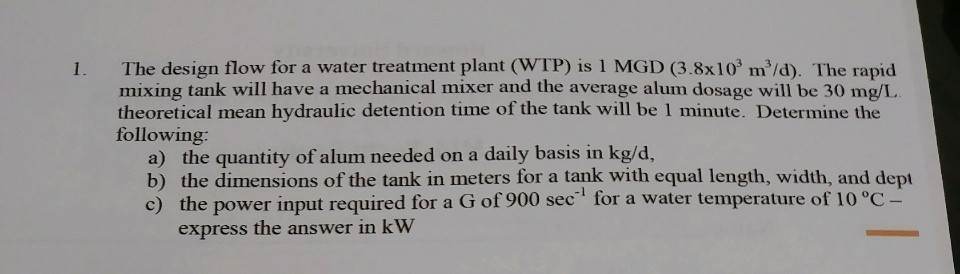1. The design flow for a water treatment plant (WTP) is 1 MGD (3.8x103 m'/d). The rapid mixing tank will have a mechanical mixer and the average alum dosage will be 30 mg/L theoretical mean hydraulic detention time of the tank will be 1 minute. Determine the following: a) the quantity of alum needed on a daily basis in kg/d, b) the dimensions of the tank in meters for a tank with equal length, width, and dept c) the power...

• ### QUESTION 4 horizontal flow grit chamber with rectangular x-section for treating maximum sewage flow of 10 MIld. Assu...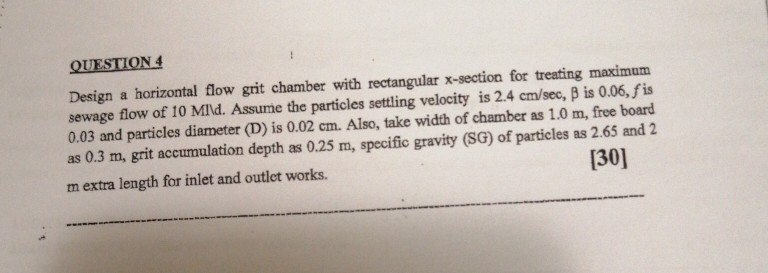QUESTION 4 horizontal flow grit chamber with rectangular x-section for treating maximum sewage flow of 10 MIld. Assume the particles settling velocity is 2.4 cm/sec, B is 0.06, fis 0.03 and particles diameter (D) is 0.02 cm. Also, take width of chamber as 1.0 m, free board as 0.3 m, grit accumulation depth as 0.25 m, specific gravity (SG) of particles as 2.65 and 2  Design a mextra length for inlet and outlet works. QUESTION 4 horizontal flow grit...

• ### You are hired to design a rectangular sedimentation tank (length to width ratio = 3:1 and...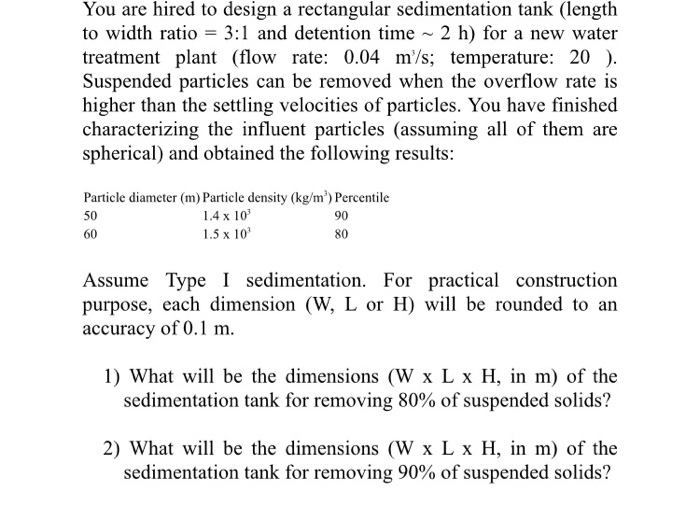You are hired to design a rectangular sedimentation tank (length to width ratio = 3:1 and detention time ~ 2 h) for a new water treatment plant (flow rate: 0.04 m/s; temperature: 20 ). Suspended particles can be removed when the overflow rate is higher than the settling velocities of particles. You have finished characterizing the influent particles (assuming all of them are spherical) and obtained the following results: Particle diameter (m) Particle density (kg/m) Percentile 50 1.4 x 10...

• ### You are hired to design a rectangular sedimentation tank (length to width ratio = 3:1 and...

You are hired to design a rectangular sedimentation tank (length to width ratio = 3:1 and detention time ~ 2 h) for a new water treatment plant (flow rate: 0.04 m3/s; temperature: 20 ℃). Suspended particles can be removed when the overflow rate is higher than the settling velocities of particles. You have finished characterizing the influent particles (assuming all of them are spherical) and obtained the following results: Particle diameter (m) Particle density (kg/m3) Percentile 50 1.4 x 103...

• ### Using the values provided in Table 21-1, minimum sewage elevation in interceptor of 112.60 m, maximum...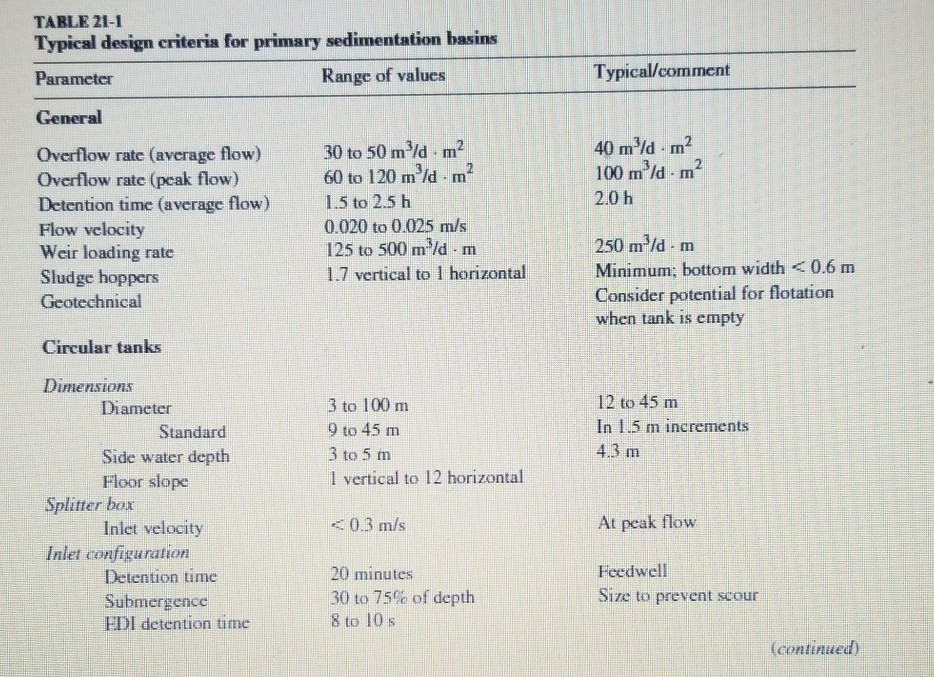Using the values provided in Table 21-1, minimum sewage elevation in interceptor of 112.60 m, maximum sewage elevation in interceptor of 112.80 m, discharge elevation to stilling well of 116.40 m, an average design flow of 8,600 m3/d, peaking factor for peak hour of 2.8 and minimum factor of 0.49. A. Determine the most efficient screw pump diameter (in m) B. How many flights are recommended C. How many pumps are recommended in the final design? Typical/comment TABLE 21-1 Typical...

• ### You are hired to design a rectangular sedimentation tank (length to width ratio = 3:1 and...

You are hired to design a rectangular sedimentation tank (length to width ratio = 3:1 and detention time ~ 2 h) for a new water treatment plant (flow rate: 0.04 m3/s; temperature: 20 ℃). Suspended particles can be removed when the overflow rate is higher than the settling velocities of particles. You have finished characterizing the influent particles (assuming all of them are spherical) and obtained the following results: Particle diameter (μm) Particle density (kg/m3) Percentile ≥ 50 ≥ 1.4...